## Sound Interference and Resonance: Standing Waves in Air Columns

### Learning Objectives

By the end of this section, you will be able to:

• Define antinode, node, fundamental, overtones, and harmonics.
• Identify instances of sound interference in everyday situations.
• Describe how sound interference occurring inside open and closed tubes changes the characteristics of the sound, and how this applies to sounds produced by musical instruments.
• Calculate the length of a tube using sound wave measurements.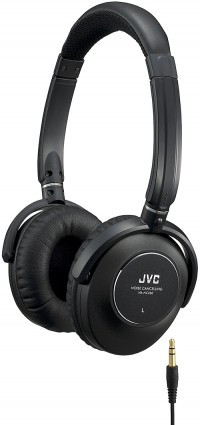Figure 1. Some types of headphones use the phenomena of constructive and destructive interference to cancel out outside noises. (credit: JVC America, Flickr)

Interference is the hallmark of waves, all of which exhibit constructive and destructive interference exactly analogous to that seen for water waves. In fact, one way to prove something “is a wave” is to observe interference effects. So, sound being a wave, we expect it to exhibit interference; we have already mentioned a few such effects, such as the beats from two similar notes played simultaneously.

Figure 2 shows a clever use of sound interference to cancel noise. Larger-scale applications of active noise reduction by destructive interference are contemplated for entire passenger compartments in commercial aircraft. To obtain destructive interference, a fast electronic analysis is performed, and a second sound is introduced with its maxima and minima exactly reversed from the incoming noise. Sound waves in fluids are pressure waves and consistent with Pascal’s principle; pressures from two different sources add and subtract like simple numbers; that is, positive and negative gauge pressures add to a much smaller pressure, producing a lower-intensity sound. Although completely destructive interference is possible only under the simplest conditions, it is possible to reduce noise levels by 30 dB or more using this technique.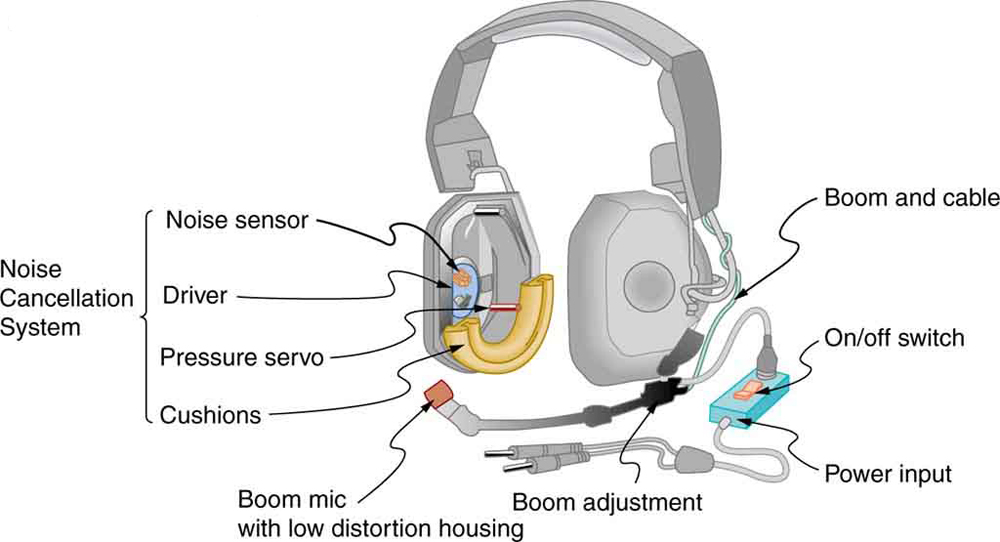Figure 2. Headphones designed to cancel noise with destructive interference create a sound wave exactly opposite to the incoming sound. These headphones can be more effective than the simple passive attenuation used in most ear protection. Such headphones were used on the record-setting, around the world nonstop flight of the Voyager aircraft to protect the pilots’ hearing from engine noise.

Where else can we observe sound interference? All sound resonances, such as in musical instruments, are due to constructive and destructive interference. Only the resonant frequencies interfere constructively to form standing waves, while others interfere destructively and are absent. From the toot made by blowing over a bottle, to the characteristic flavor of a violin’s sounding box, to the recognizability of a great singer’s voice, resonance and standing waves play a vital role.

### Interference

Interference is such a fundamental aspect of waves that observing interference is proof that something is a wave. The wave nature of light was established by experiments showing interference. Similarly, when electrons scattered from crystals exhibited interference, their wave nature was confirmed to be exactly as predicted by symmetry with certain wave characteristics of light.

Suppose we hold a tuning fork near the end of a tube that is closed at the other end, as shown in Figure 3, Figure 4, Figure 5, and Figure 6. If the tuning fork has just the right frequency, the air column in the tube resonates loudly, but at most frequencies it vibrates very little. This observation just means that the air column has only certain natural frequencies. The figures show how a resonance at the lowest of these natural frequencies is formed. A disturbance travels down the tube at the speed of sound and bounces off the closed end. If the tube is just the right length, the reflected sound arrives back at the tuning fork exactly half a cycle later, and it interferes constructively with the continuing sound produced by the tuning fork. The incoming and reflected sounds form a standing wave in the tube as shown.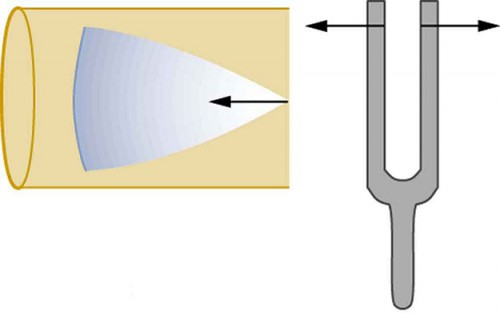Figure 3. Resonance of air in a tube closed at one end, caused by a tuning fork. A disturbance moves down the tube.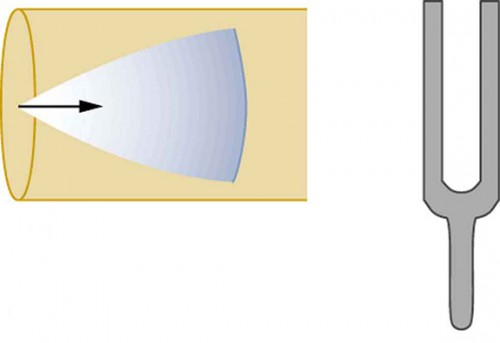Figure 4. Resonance of air in a tube closed at one end, caused by a tuning fork. The disturbance reflects from the closed end of the tube.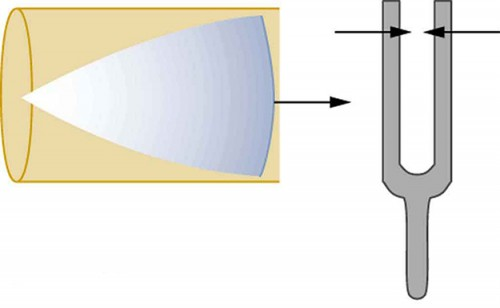Figure 5. Resonance of air in a tube closed at one end, caused by a tuning fork. If the length of the tube L is just right, the disturbance gets back to the tuning fork half a cycle later and interferes constructively with the continuing sound from the tuning fork. This interference forms a standing wave, and the air column resonates.Figure 6. Resonance of air in a tube closed at one end, caused by a tuning fork. A graph of air displacement along the length of the tube shows none at the closed end, where the motion is constrained, and a maximum at the open end. This standing wave has one-fourth of its wavelength in the tube, so that λ = 4L.

The standing wave formed in the tube has its maximum air displacement (an antinode) at the open end, where motion is unconstrained, and no displacement (a node) at the closed end, where air movement is halted. The distance from a node to an antinode is one-fourth of a wavelength, and this equals the length of the tube; thus, λ = 4L. This same resonance can be produced by a vibration introduced at or near the closed end of the tube, as shown in Figure 7. It is best to consider this a natural vibration of the air column independently of how it is induced.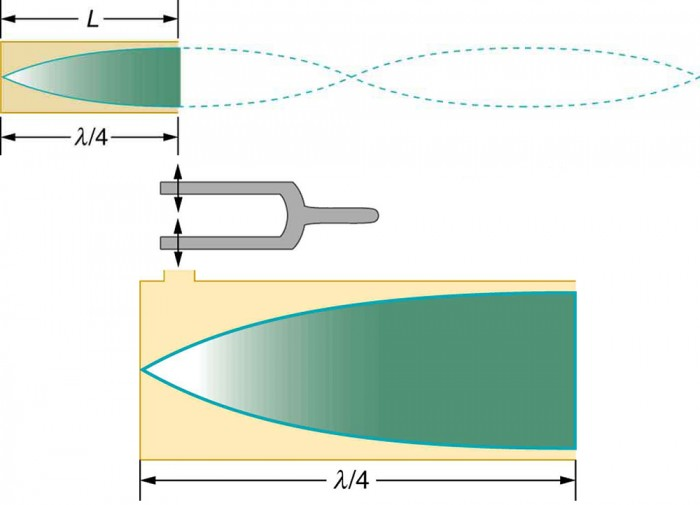Figure 7. The same standing wave is created in the tube by a vibration introduced near its closed end.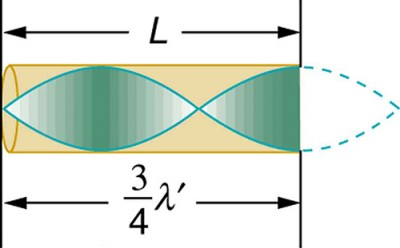Figure 8. Another resonance for a tube closed at one end. This has maximum air displacements at the open end, and none at the closed end. The wavelength is shorter, with three-fourths λ′ equaling the length of the tube, so that $\lambda^{\prime}=\frac{4L}{3}\\$. This higher-frequency vibration is the first overtone.

Given that maximum air displacements are possible at the open end and none at the closed end, there are other, shorter wavelengths that can resonate in the tube, such as the one shown in Figure 8. Here the standing wave has three-fourths of its wavelength in the tube, or $L=\frac{3}{4}\lambda^{\prime}\\$, so that $\lambda^{\prime}=\frac{4L}{3}\\$. Continuing this process reveals a whole series of shorter-wavelength and higher-frequency sounds that resonate in the tube. We use specific terms for the resonances in any system. The lowest resonant frequency is called the fundamental, while all higher resonant frequencies are called overtones. All resonant frequencies are integral multiples of the fundamental, and they are collectively called harmonics. The fundamental is the first harmonic, the first overtone is the second harmonic, and so on. Figure 9 shows the fundamental and the first three overtones (the first four harmonics) in a tube closed at one end.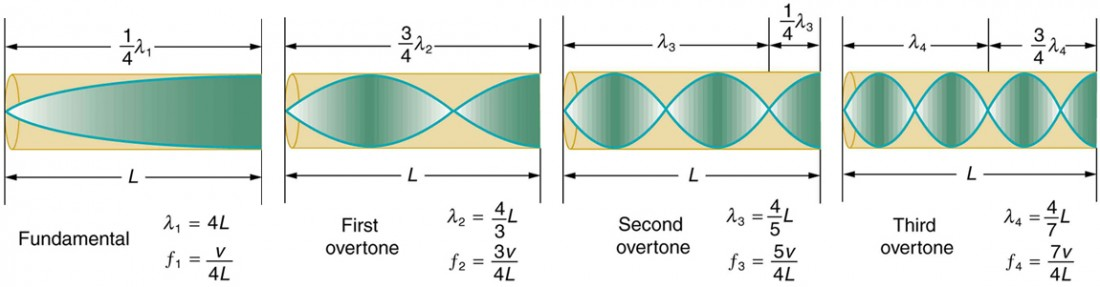Figure 9. The fundamental and three lowest overtones for a tube closed at one end. All have maximum air displacements at the open end and none at the closed end.

The fundamental and overtones can be present simultaneously in a variety of combinations. For example, middle C on a trumpet has a sound distinctively different from middle C on a clarinet, both instruments being modified versions of a tube closed at one end. The fundamental frequency is the same (and usually the most intense), but the overtones and their mix of intensities are different and subject to shading by the musician. This mix is what gives various musical instruments (and human voices) their distinctive characteristics, whether they have air columns, strings, sounding boxes, or drumheads. In fact, much of our speech is determined by shaping the cavity formed by the throat and mouth and positioning the tongue to adjust the fundamental and combination of overtones. Simple resonant cavities can be made to resonate with the sound of the vowels, for example. (See Figure 10.) In boys, at puberty, the larynx grows and the shape of the resonant cavity changes giving rise to the difference in predominant frequencies in speech between men and women.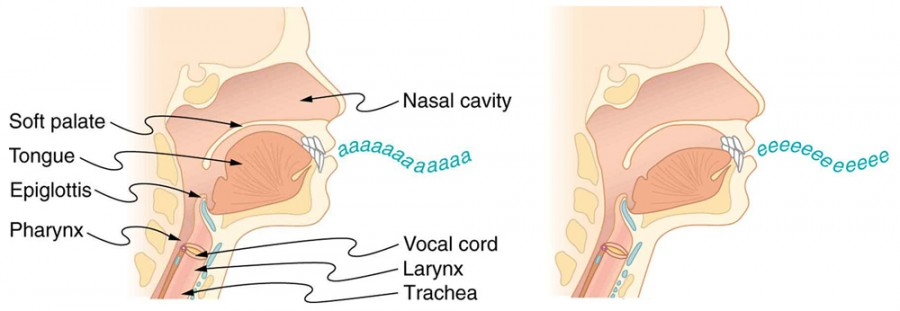Figure 10. The throat and mouth form an air column closed at one end that resonates in response to vibrations in the voice box. The spectrum of overtones and their intensities vary with mouth shaping and tongue position to form different sounds. The voice box can be replaced with a mechanical vibrator, and understandable speech is still possible. Variations in basic shapes make different voices recognizable.

Now let us look for a pattern in the resonant frequencies for a simple tube that is closed at one end. The fundamental has λ = 4L, and frequency is related to wavelength and the speed of sound as given by vw = fλ.

Solving for f in this equation gives

$f=\frac{v_{\text{w}}}{\lambda}=\frac{v_{\text{w}}}{4L}\\$,

where vw is the speed of sound in air. Similarly, the first overtone has $\lambda^{\prime}=\frac{4L}{3}\\$ (see Figure 9), so that

$f^{\prime}=3\frac{v_{\text{w}}}{4L}=3f\\$.

Because f′ = 3f, we call the first overtone the third harmonic. Continuing this process, we see a pattern that can be generalized in a single expression. The resonant frequencies of a tube closed at one end are

$f_n=n\frac{v_{\text{w}}}{4L},n=1,3,5\\$,

where f1 is the fundamental, f3 is the first overtone, and so on. It is interesting that the resonant frequencies depend on the speed of sound and, hence, on temperature. This dependence poses a noticeable problem for organs in old unheated cathedrals, and it is also the reason why musicians commonly bring their wind instruments to room temperature before playing them.

### Example 1. Find the Length of a Tube with a 128 Hz Fundamental

1. What length should a tube closed at one end have on a day when the air temperature, is 22.0ºC, if its fundamental frequency is to be 128 Hz (C below middle C)?
2. What is the frequency of its fourth overtone?

#### Strategy

The length L can be found from the relationship in $f_n=n\frac{v_{\text{w}}}{4L}\\$, but we will first need to find the speed of sound vw.

#### Solution for Part 1

Identify knowns:

• the fundamental frequency is 128 Hz
• the air temperature is 22.0ºC

Use $f_n=n\frac{v_{\text{w}}}{4L}\\$ to find the fundamental frequency (= 1):

$f_1=\frac{v_{\text{w}}}{4L}\\$

Solve this equation for length: $L=\frac{v_{\text{w}}}{4f_1}\\$.

Find the speed of sound using $v_{\text{w}}=\left(331\text{ m/s}\right)\sqrt{\frac{T}{273\text{ K}}}\\$.

$v_{\text{w}}=\left(331\text{ m/s}\right)\sqrt{\frac{295\text{ K}}{273\text{ K}}}=344\text{ m/s}\\$

Enter the values of the speed of sound and frequency into the expression for L.

$L=\frac{v_{\text{w}}}{4f_1}=\frac{344\text{ m/s}}{4(128\text{ Hz})}=0.672\text{ m}\\$

#### Discussion on Part 1

Many wind instruments are modified tubes that have finger holes, valves, and other devices for changing the length of the resonating air column and hence, the frequency of the note played. Horns producing very low frequencies, such as tubas, require tubes so long that they are coiled into loops.

#### Solution for Part 2

Identify knowns:

• the first overtone has = 3
• the second overtone has = 5
• the third overtone has = 7
• the fourth overtone has = 9

Enter the value for the fourth overtone into $f_n=n\frac{v_{\text{w}}}{4L}\\$:

$f_9=9\frac{v_{\text{w}}}{4L}=9f_1=1.15\text{ kHz}\\$

#### Discussion on Part 2

Whether this overtone occurs in a simple tube or a musical instrument depends on how it is stimulated to vibrate and the details of its shape. The trombone, for example, does not produce its fundamental frequency and only makes overtones.

Another type of tube is one that is open at both ends. Examples are some organ pipes, flutes, and oboes. The resonances of tubes open at both ends can be analyzed in a very similar fashion to those for tubes closed at one end. The air columns in tubes open at both ends have maximum air displacements at both ends, as illustrated in Figure 11. Standing waves form as shown.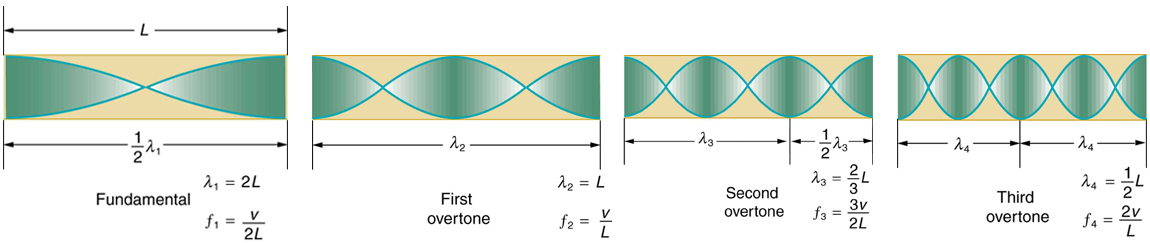Figure 11. The resonant frequencies of a tube open at both ends are shown, including the fundamental and the first three overtones. In all cases the maximum air displacements occur at both ends of the tube, giving it different natural frequencies than a tube closed at one end.

Based on the fact that a tube open at both ends has maximum air displacements at both ends, and using Figure 11 as a guide, we can see that the resonant frequencies of a tube open at both ends are:

$f_n=n\frac{v_{\text{w}}}{2L},n=1,2,3,\dots,\\$

where f1 is the fundamental, f2 is the first overtone, f3 is the second overtone, and so on. Note that a tube open at both ends has a fundamental frequency twice what it would have if closed at one end. It also has a different spectrum of overtones than a tube closed at one end. So if you had two tubes with the same fundamental frequency but one was open at both ends and the other was closed at one end, they would sound different when played because they have different overtones. Middle C, for example, would sound richer played on an open tube, because it has even multiples of the fundamental as well as odd. A closed tube has only odd multiples.

### Real-World Applications: Resonance in Everyday Systems

Resonance occurs in many different systems, including strings, air columns, and atoms. Resonance is the driven or forced oscillation of a system at its natural frequency. At resonance, energy is transferred rapidly to the oscillating system, and the amplitude of its oscillations grows until the system can no longer be described by Hooke’s law. An example of this is the distorted sound intentionally produced in certain types of rock music.Figure 12. String instruments such as violins and guitars use resonance in their sounding boxes to amplify and enrich the sound created by their vibrating strings. The bridge and supports couple the string vibrations to the sounding boxes and air within. (credits: guitar, Feliciano Guimares, Fotopedia; violin, Steve Snodgrass, Flickr)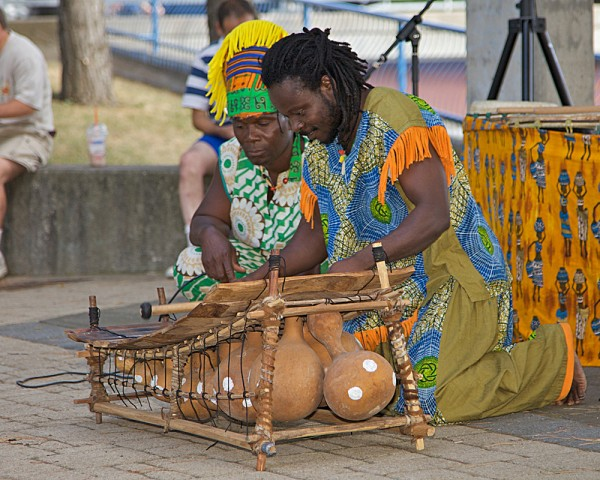Figure 13. Resonance has been used in musical instruments since prehistoric times. This marimba uses gourds as resonance chambers to amplify its sound. (credit: APC Events, Flickr)

Wind instruments use resonance in air columns to amplify tones made by lips or vibrating reeds. Other instruments also use air resonance in clever ways to amplify sound. Figure 12 shows a violin and a guitar, both of which have sounding boxes but with different shapes, resulting in different overtone structures. The vibrating string creates a sound that resonates in the sounding box, greatly amplifying the sound and creating overtones that give the instrument its characteristic flavor. The more complex the shape of the sounding box, the greater its ability to resonate over a wide range of frequencies. The marimba, like the one shown in Figure 13 uses pots or gourds below the wooden slats to amplify their tones. The resonance of the pot can be adjusted by adding water.

We have emphasized sound applications in our discussions of resonance and standing waves, but these ideas apply to any system that has wave characteristics. Vibrating strings, for example, are actually resonating and have fundamentals and overtones similar to those for air columns. More subtle are the resonances in atoms due to the wave character of their electrons. Their orbitals can be viewed as standing waves, which have a fundamental (ground state) and overtones (excited states). It is fascinating that wave characteristics apply to such a wide range of physical systems.

#### Part 1

Describe how noise-canceling headphones differ from standard headphones used to block outside sounds.

##### Solution

Regular headphones only block sound waves with a physical barrier. Noise-canceling headphones use destructive interference to reduce the loudness of outside sounds.

#### Part 2

How is it possible to use a standing wave’s node and antinode to determine the length of a closed-end tube?

##### Solution

When the tube resonates at its natural frequency, the wave’s node is located at the closed end of the tube, and the antinode is located at the open end. The length of the tube is equal to one-fourth of the wavelength of this wave. Thus, if we know the wavelength of the wave, we can determine the length of the tube.

## PhET Explorations: Sound

This simulation lets you see sound waves. Adjust the frequency or volume and you can see and hear how the wave changes. Move the listener around and hear what she hears.

## Section Summary

• Sound interference and resonance have the same properties as defined for all waves.
• In air columns, the lowest-frequency resonance is called the fundamental, whereas all higher resonant frequencies are called overtones. Collectively, they are called harmonics.
• The resonant frequencies of a tube closed at one end are: ${f}_{n}=n\frac{{v}_{w}}{4L}\text{, }n=1, 3, 5\dots\\$, f1 is the fundamental and L is the length of the tube.
• The resonant frequencies of a tube open at both ends are: ${f}_{n}=n\frac{{v}_{w}}{2L}\text{, }n=1, 2, 3\dots\\$

### Conceptual Questions

1. How does an unamplified guitar produce sounds so much more intense than those of a plucked string held taut by a simple stick?
2. You are given two wind instruments of identical length. One is open at both ends, whereas the other is closed at one end. Which is able to produce the lowest frequency?
3. What is the difference between an overtone and a harmonic? Are all harmonics overtones? Are all overtones harmonics?

### Problems & Exercises

1. A “showy” custom-built car has two brass horns that are supposed to produce the same frequency but actually emit 263.8 and 264.5 Hz. What beat frequency is produced?

2. What beat frequencies will be present: (a) If the musical notes A and C are played together (frequencies of 220 and 264 Hz)? (b) If D and F are played together (frequencies of 297 and 352 Hz)? (c) If all four are played together?

3. What beat frequencies result if a piano hammer hits three strings that emit frequencies of 127.8, 128.1, and 128.3 Hz?

4. A piano tuner hears a beat every 2.00 s when listening to a 264.0-Hz tuning fork and a single piano string. What are the two possible frequencies of the string?

5. (a) What is the fundamental frequency of a 0.672-m-long tube, open at both ends, on a day when the speed of sound is 344 m/s? (b) What is the frequency of its second harmonic?

6. If a wind instrument, such as a tuba, has a fundamental frequency of 32.0 Hz, what are its first three overtones? It is closed at one end. (The overtones of a real tuba are more complex than this example, because it is a tapered tube.)

7. What are the first three overtones of a bassoon that has a fundamental frequency of 90.0 Hz? It is open at both ends. (The overtones of a real bassoon are more complex than this example, because its double reed makes it act more like a tube closed at one end.)

8. How long must a flute be in order to have a fundamental frequency of 262 Hz (this frequency corresponds to middle C on the evenly tempered chromatic scale) on a day when air temperature is 20.0ºC? It is open at both ends.

9. What length should an oboe have to produce a fundamental frequency of 110 Hz on a day when the speed of sound is 343 m/s? It is open at both ends.

10. What is the length of a tube that has a fundamental frequency of 176 Hz and a first overtone of 352 Hz if the speed of sound is 343 m/s?

11. (a) Find the length of an organ pipe closed at one end that produces a fundamental frequency of 256 Hz when air temperature is 18.0ºC. (b) What is its fundamental frequency at 25.0ºC?

12. By what fraction will the frequencies produced by a wind instrument change when air temperature goes from 10.0ºC to 30.0ºC? That is, find the ratio of the frequencies at those temperatures.

13. The ear canal resonates like a tube closed at one end. (See Figure 5 in Hearing.) If ear canals range in length from 1.80 to 2.60 cm in an average population, what is the range of fundamental resonant frequencies? Take air temperature to be 37.0ºC, which is the same as body temperature. How does this result correlate with the intensity versus frequency graph of the human ear (Figure 14)?Figure 14. The shaded region represents frequencies and intensity levels found in normal conversational speech. The 0-phon line represents the normal hearing threshold, while those at 40 and 60 represent thresholds for people with 40- and 60-phon hearing losses, respectively.

14. Calculate the first overtone in an ear canal, which resonates like a 2.40-cm-long tube closed at one end, by taking air temperature to be 37.0ºC. Is the ear particularly sensitive to such a frequency? (The resonances of the ear canal are complicated by its nonuniform shape, which we shall ignore.)

15. A crude approximation of voice production is to consider the breathing passages and mouth to be a resonating tube closed at one end. (See Figure 10.) (a) What is the fundamental frequency if the tube is 0.240-m long, by taking air temperature to be 37.0ºC? (b) What would this frequency become if the person replaced the air with helium? Assume the same temperature dependence for helium as for air.

16. (a) Students in a physics lab are asked to find the length of an air column in a tube closed at one end that has a fundamental frequency of 256 Hz. They hold the tube vertically and fill it with water to the top, then lower the water while a 256-Hz tuning fork is rung and listen for the first resonance. What is the air temperature if the resonance occurs for a length of 0.336 m? (b) At what length will they observe the second resonance (first overtone)?

17. What frequencies will a 1.80-m-long tube produce in the audible range at 20.0ºC if: (a) The tube is closed at one end? (b) It is open at both ends?

## Glossary

antinode: point of maximum displacement

node: point of zero displacement

fundamental: the lowest-frequency resonance

overtones: all resonant frequencies higher than the fundamental

harmonics: the term used to refer collectively to the fundamental and its overtones

### Selected Solutions to Problems & Exercises

1. 0.7 Hz

3. 0.3 Hz, 0.2 Hz, 0.5 Hz

5. (a) 256 Hz; (b) 512 Hz

7. 180 Hz, 270 Hz, 360 Hz

9. 1.56 m

11. (a) 0.334 m; (b) 259 Hz

13. 3.39 to 4.90 kHz

15. (a) 367 Hz; (b) 1.07 kHz

17. (a) fn = n(47.6 Hz), = 1, 3, 5,…, 419; (b) fn = n(95.3 Hz), = 1, 2, 3,…, 210